# COMBINED BENDING, DIRECT AND TORSIONAL STRESSESReading time: 1 minute

COMBINED BENDING, DIRECT AND TORSIONAL STRESSES IN SHAFTS

Cases arise such as in propeller shafts of ships where a shaft is subjected to direct thrust in addition to bending moment and torsion. In such cases the direct stresses due to bending moment and the axial thrust have to be combined into a single resultant.

a) Direct stress,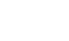where P = axial thrust

A = area of cross-section

b) Bending stress,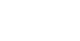where M = bending moment

y- distance of fibre from neutral axis

I = moment of inertia.

c) Torsional Stress (shear),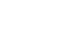where T = torque

J = polar moment of inertia

Combined Stresses:

Under combination of these stresses,

(d) Principle Stress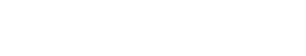(e) Maximum shear stress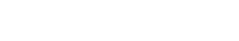Equivalent Moment and Torque

Equivalent bending moment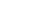may be defined as the bending moment which will produce the same direct stress as produced by the bending moment and torque acting separately. Similarly, the equivalent torque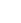may be defined as the torque which wil produce the same maximum shear stress as produced by the bending moment and torque acting separately.

For a circular shaft of diameter, d = 2r(f)(g)If P = 0

(h)Power transmitted by a shaft

Torque =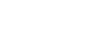Where power is measured in watts and n is the speed in rpm.